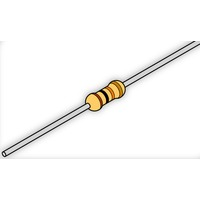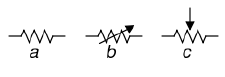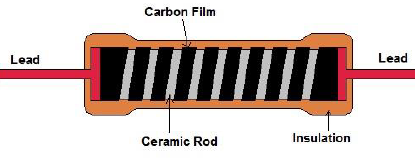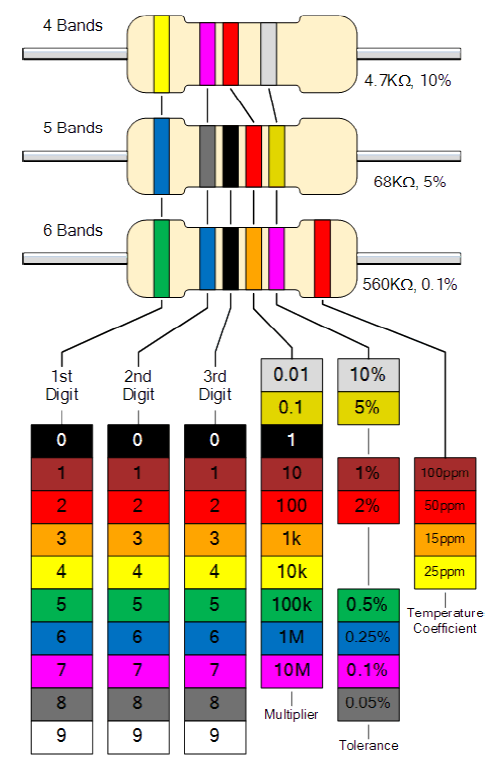# What is a Resistor?

Author: Tyson Popynick   Date Posted:6 April 2016What is a resistor?
A resistor is an analog component that acts to lower both voltage and current levels in a circuit.

What is the schematic symbol for a resistor?
These are the commonly used symbols for resistors. These are the US representations, which are the most commonly used in my experience.

A) Fixed value resistor
B) Variable value resistor
C) Potentiometer

NOTE: There IS a big difference between a variable resistor and a potentiometer. A variable resistor is a component which can have its resistance changed, whereas a potentiometer is essentially a voltage divider circuit in a component.Image by Scwerllguy - Own work, CC BY-SA 3.0

How do I tell its value?
Resistors have bands of colors on them. It is quite easy to see these with a magnifying glass in a well-lit room, although they can be a bit difficult to see sometimes. We use a color chart as shown later in this guide, to work out the values based on the bands. A great alternative is to use the resistance setting on your multimeter to get the values. If your meter supports it, you will see a Ω on the interface. Remember you cannot use this to test resistors that are part of a circuit, as the value will be thrown off by any other components connected to the one you are testing.

What types of resistor might I use?
There are 3 main types:

• Fixed Resistor This type of resistor has a fixed resistance value
• Variable Resistor This type of resistor has a dial or screw to adjust the resistance value, such as in volume controls for instance.
• Potentiometer This is essentially a voltage divider in a component.What are some common uses?
Resistors are commonly used to adjust voltages for logic circuits (eg: 5v down to 3.3v), they are also used to limit current such as when we use a resistor along with an LED. This is to protect the Arduino digital I/O pin from damage as the LED draws more current than it can safely handle.

We can use them to build voltage divider circuits, use them as a load, we can use them to bias elements of our circuit by manipulating the resistance values at each path.

There are 2 main types of resistor: There is the fixed value resistor and there is the variable resistor. A fixed value resistor will generally look somewhat similar to A in the image above. There are also other packages, such as SMD and larger versions. However you will likely only need to worry about these larger through-hole components.

Resistors are made of a conductive material that coils around by means of ceramic spacers. This is coated in an insulator like ceramic after leads are embedded in the ends. We then use these leads to interface with the component.

How do resistors work?
Resistors are made by packing carbon or another conductive material, in a fine-powder form along with ceramic or other insulating material in a hard resin. The ratio between conductor and insulator determines the resistance value.

Example Use:
We use resistors in pretty much ever circuit we make. A great example is in applications with LEDs. We use a resistor here so that even though the LED is pulling a large enough current to damage our Arduino, the resistor will drop that current back down to tolerable levels and we will be safe.